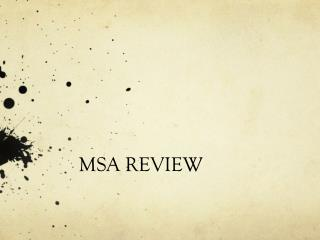DownloadDownload PresentationMSA REVIEW

MSA REVIEW

Télécharger la présentationMSA REVIEW

- - - - - - - - - - - - - - - - - - - - - - - - - - - E N D - - - - - - - - - - - - - - - - - - - - - - - - - - -
Presentation Transcript

1. MSA REVIEW

2. What is the formula used to find the area of a trapezoid?

3. What is the area of a trapezoid that has a base of 4 in, a height of 5 in and another base of 10 in?

4. Explain how to find the perimeter of any polygon.

5. What is the “secret” to adding and subtracting decimals?

6. Simplify: 123 + 12.7 + 1.245

7. What is expanded form?

8. Write 9.87 in expanded form.

9. What is the total surface area of a box that has a length of 14 in, a width of 2 in and a height of 3 in? (Draw a picture!)

10. Put these numbers in order from least to greatest. 19.05, 19.018, 19.1, 19.153 Hint: Line up the decimals.

11. What are the 3 measures of central tendency?

12. Explain how to find the: Mean Median Mode

13. What is the mode of the following data: 12, 6, 10, 8, 12, 20, 9?

14. What is the median of the following data: 12, 6, 10, 8, 12, 20, 9?

15. What are complementary angles? • What are supplementary angles?

16. Raj said, "I am thinking of two complementary angles. One of the angles measures 43°.” What is the measure of the complement?

17. Ms. Bader's purse contains 8 quarters, 3 nickels, 5 dimes, and 4 pennies. If she randomly selects one coin from her purse, what is the probability that she selects a nickel?

18. On your desk you have 5 black, 3 blue, 2 red, and 5 green pens. What is the probability of picking a blue pen without looking?

19. Anita tosses a quarter and then rolls a number cube. What is the total number of outcomes for this event?

20. How many degrees are inside of a quadrilateral?

21. A quadrilateral has the following angle measurements: 102°, 91° and 86° What is the missing angle measurement?

22. If m = 5, what is the value of (3m + 10) ÷ (9 + 16)?

23. What is the square root of 100?

24. What is 20% of 900?

25. What is the magic number for a triangle??

26. A triangle has angle measures of 90° and 27°. What is the measure of the missing angle?

27. Simplify the expression:

28. Simplify the expression:

29. Multiply: -62(17)

30. Find the value of the expression: 12 ÷ 3 + 9 – 4  6

31. Write 7 x 7 x 7 x 7 x 7 in exponential form.

32. What number is represented by the expanded form of: 100 + 20 + 0.05 + 0.0007?

33. Solve for x: 3x – 1 = 11

34. Write the fraction as a decimal and percent.

35. How do you add and subtract fractions?

36. Simplify.

37. Simplify.

38. How do you multiply fractions?

39. Simplify.

40. Simplify.

41. Simplify.

42. What is the formula used to find the area of a triangle?

43. Find the area. 14 ft 10 ft 8 ft 20 ft

44. Simplify.

45. Simplify.

46. Simplify.